# Selina Solutions Concise Maths Class 10 Chapter 18 Tangents and Intersecting Chords Exercise 18(A)

Differences between a secant and a tangent are understood and a few important theorems related to tangent and touching circles are discussed in this exercise. The Selina Solutions for Class 10 Maths is prepared for students who are looking for reference/guide to clarify doubts and learn the correct steps in solving problems. The solutions of this exercise are available in the Concise Selina Solutions for Class 10 Maths Chapter 18 Tangents and Intersecting Chords Exercise 18(A) PDF, which is provided in the link attached below.

## Selina Solutions Concise Maths Class 10 Chapter 18 Tangents and Intersecting Chords Exercise 18(A) Download PDF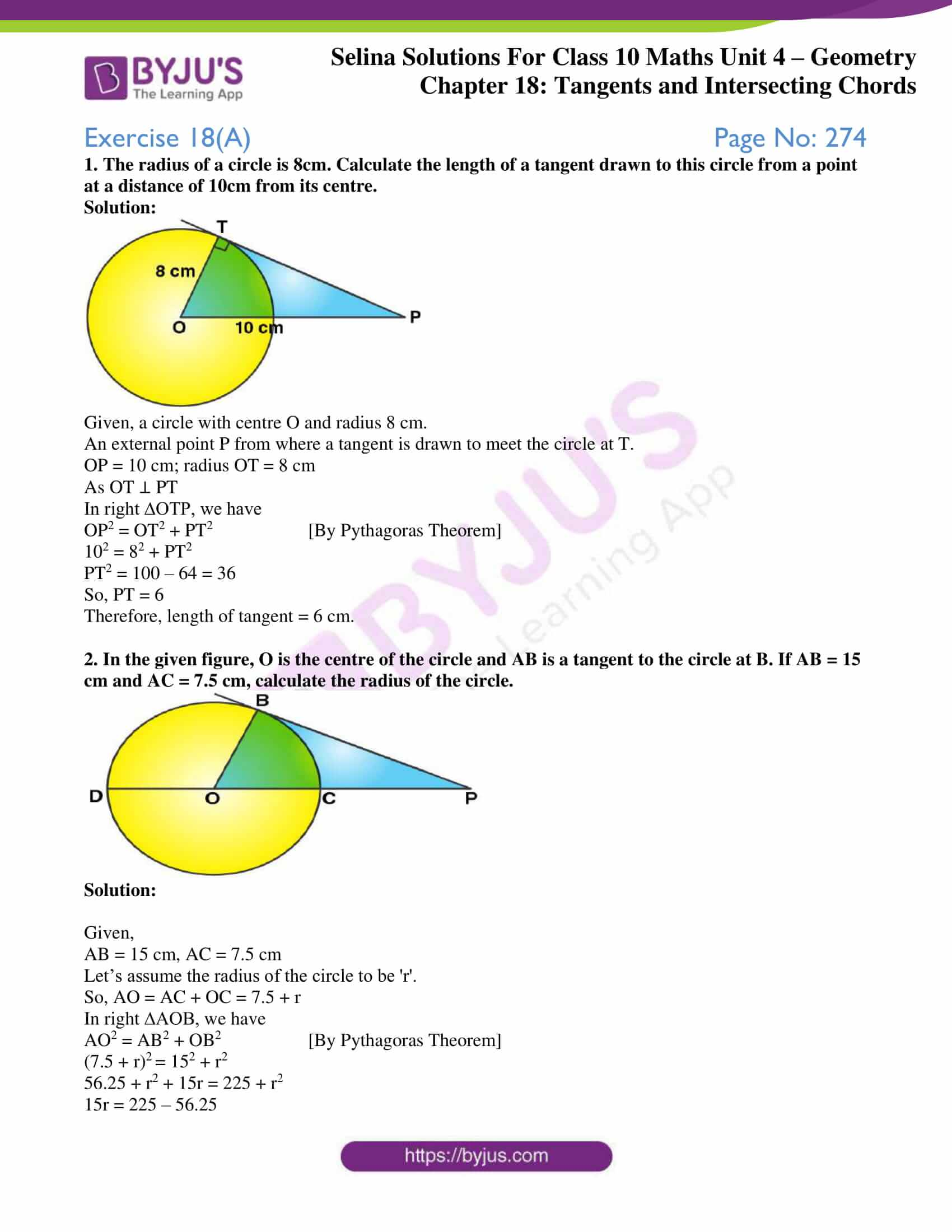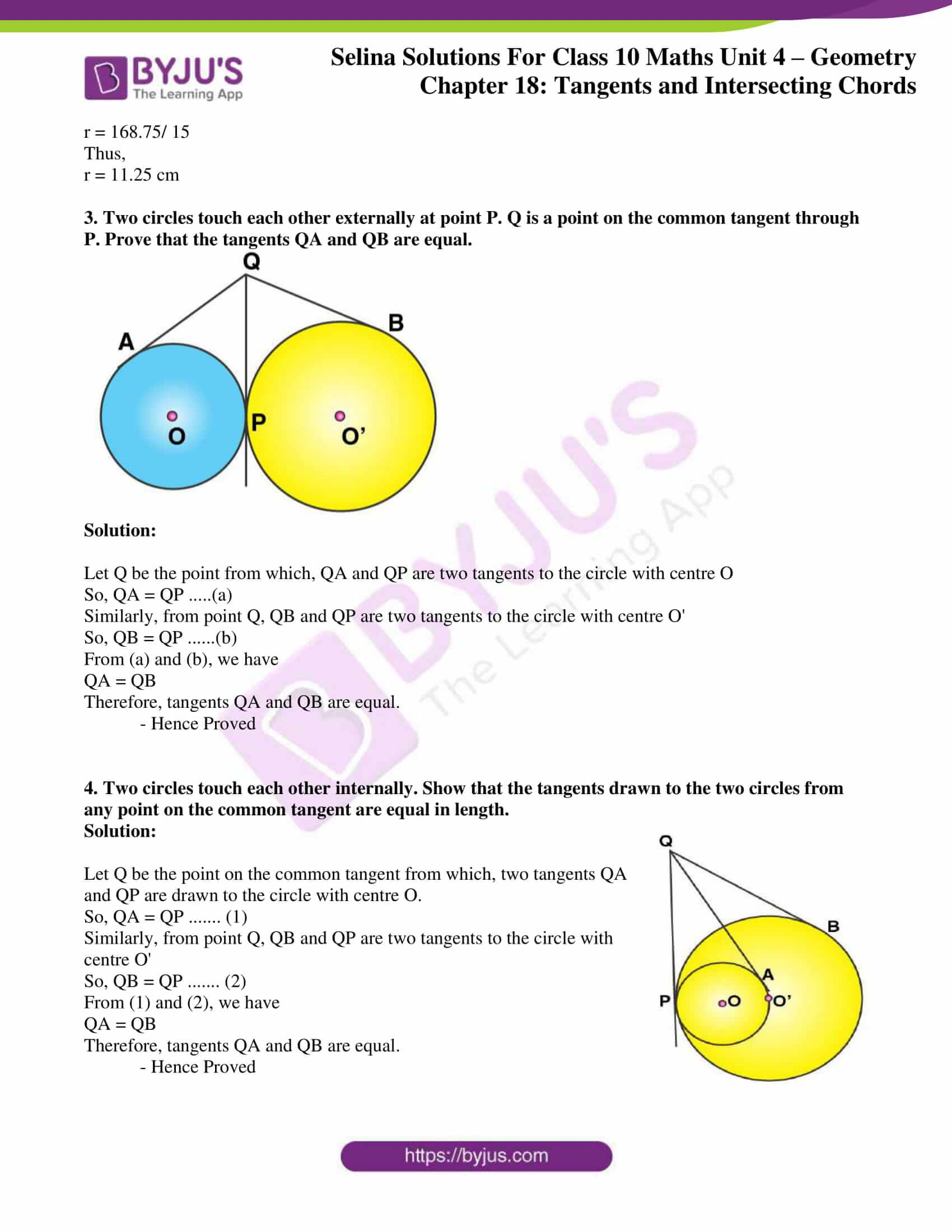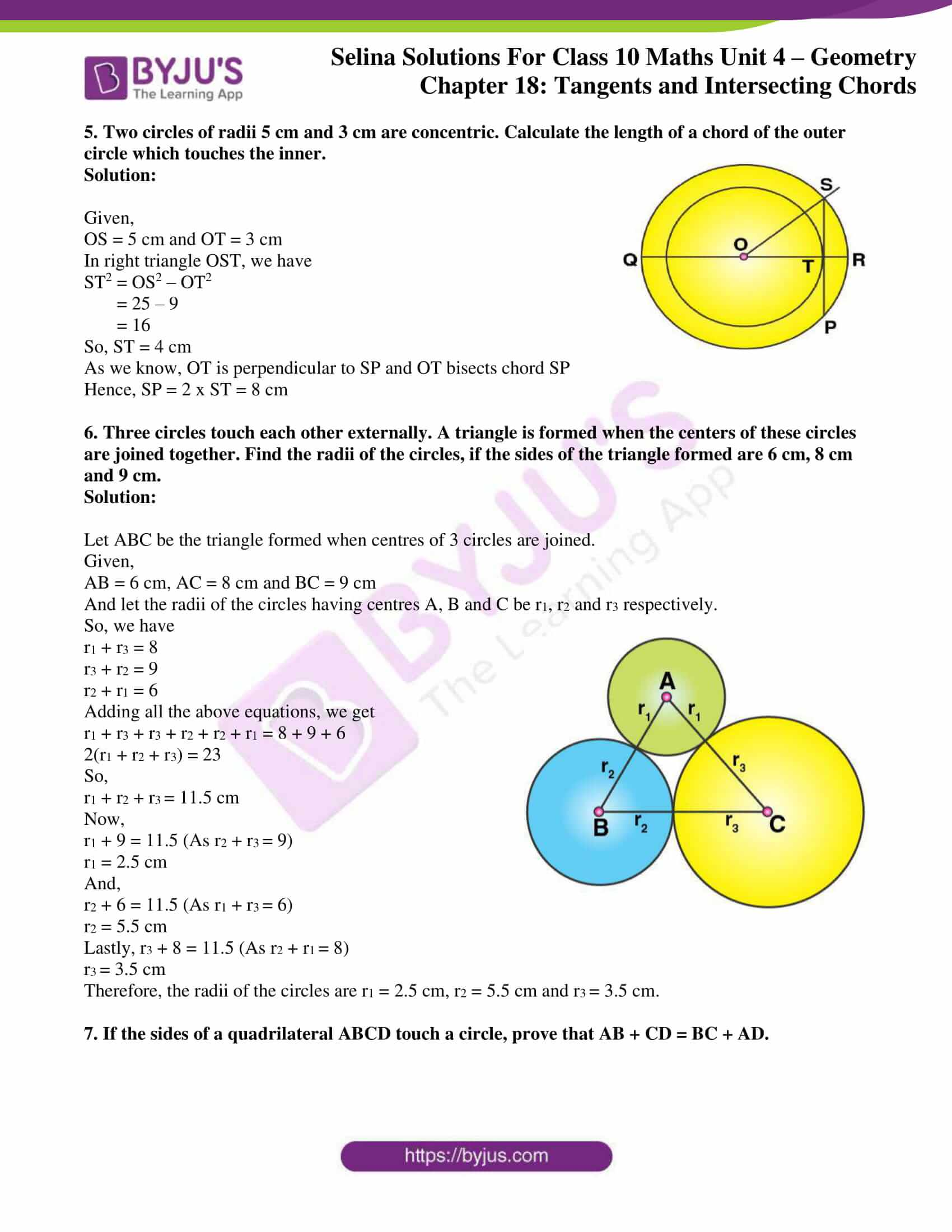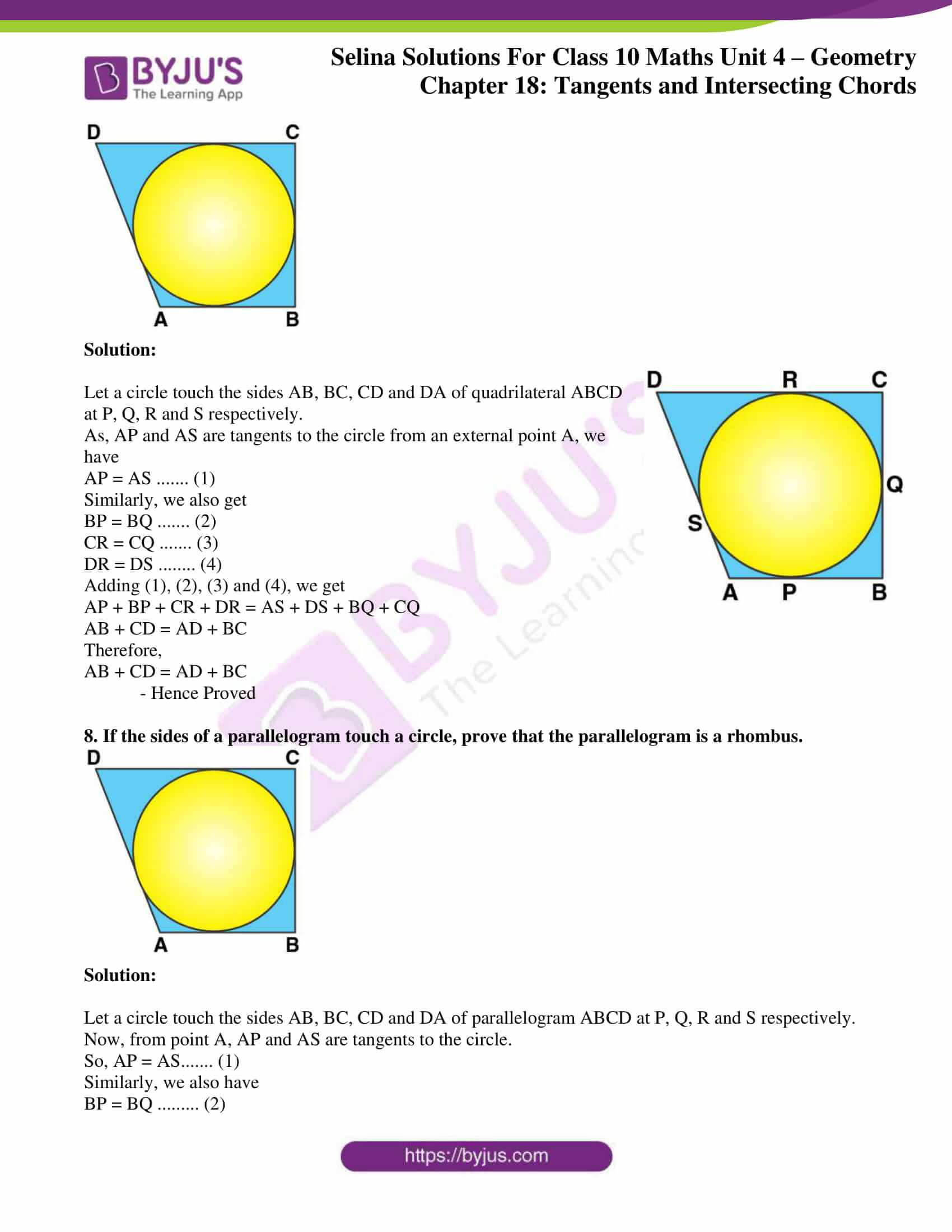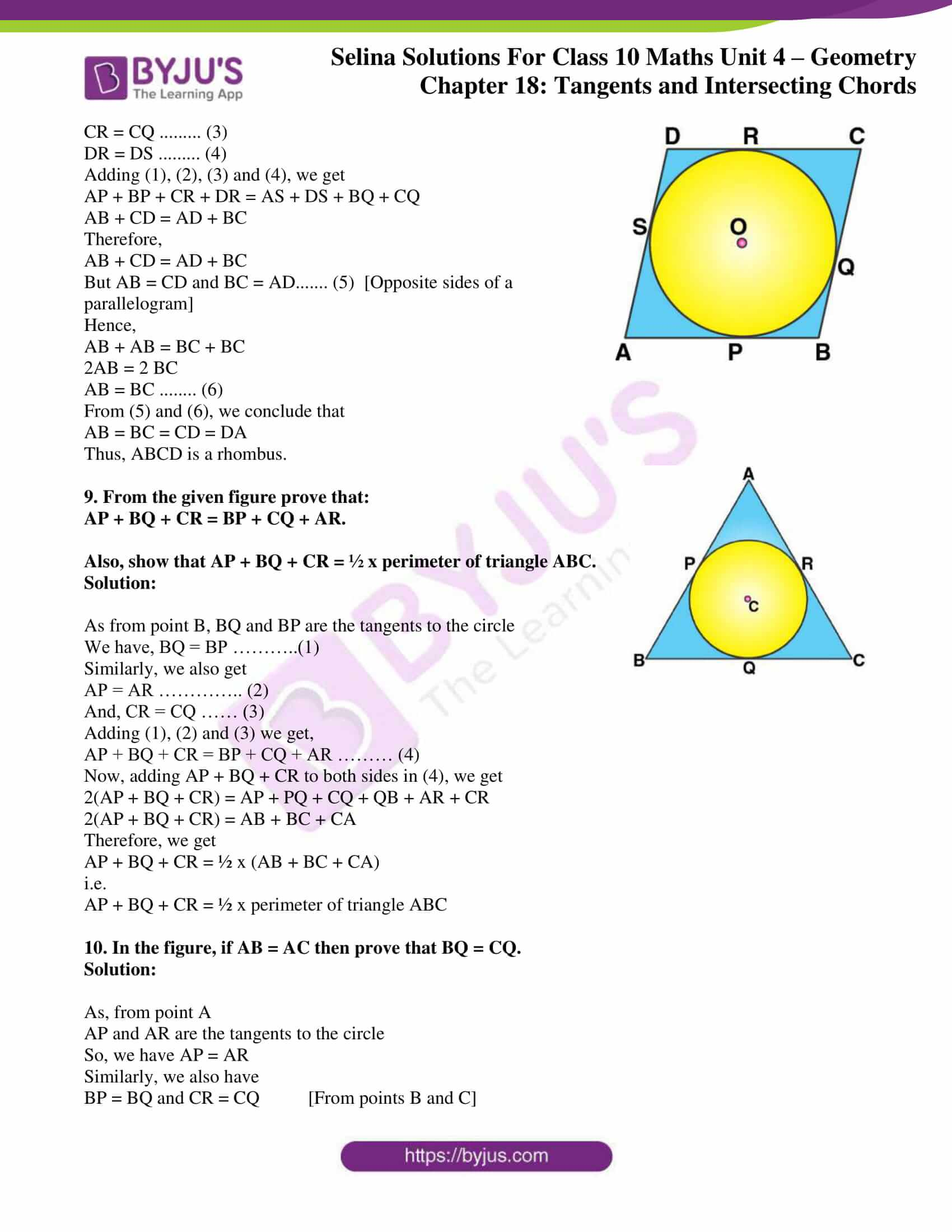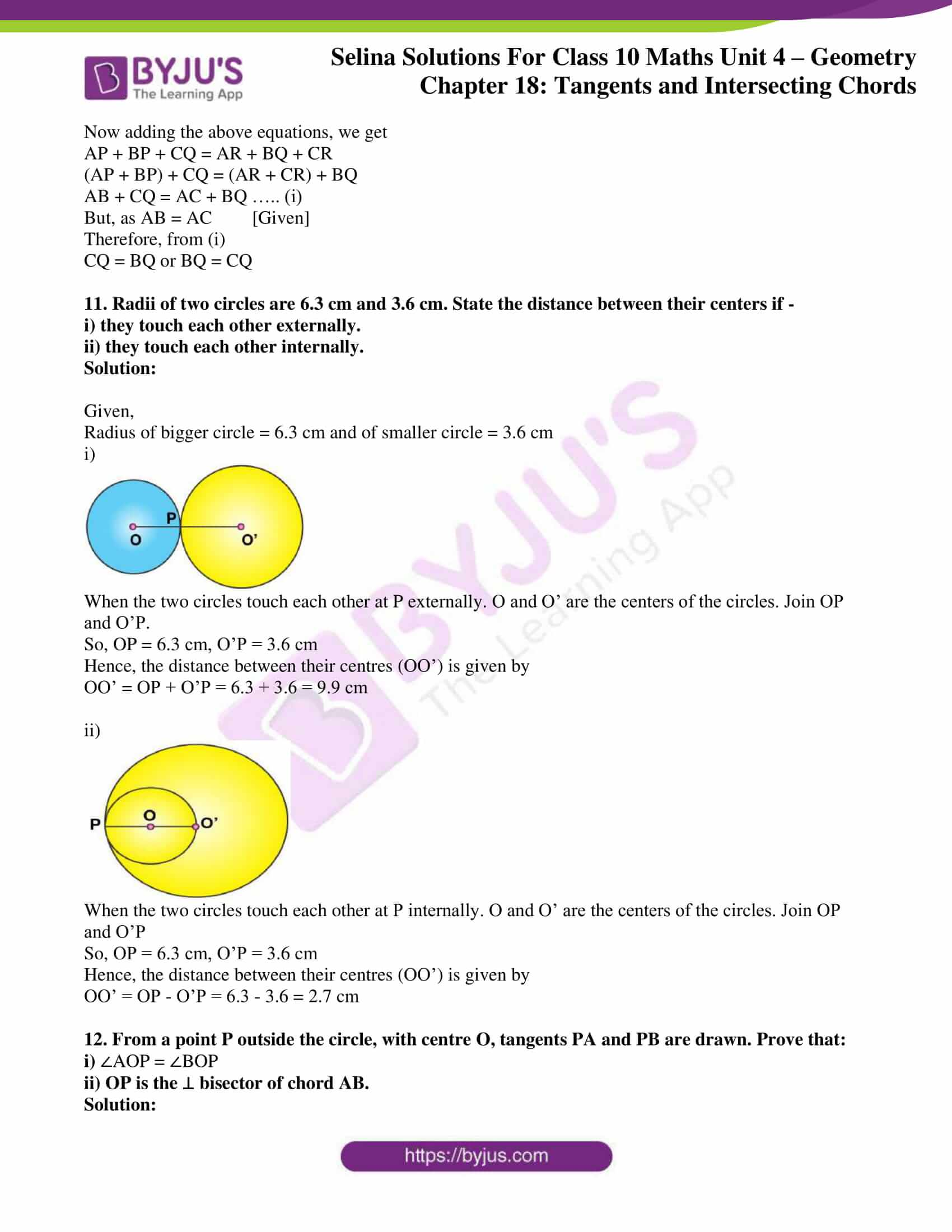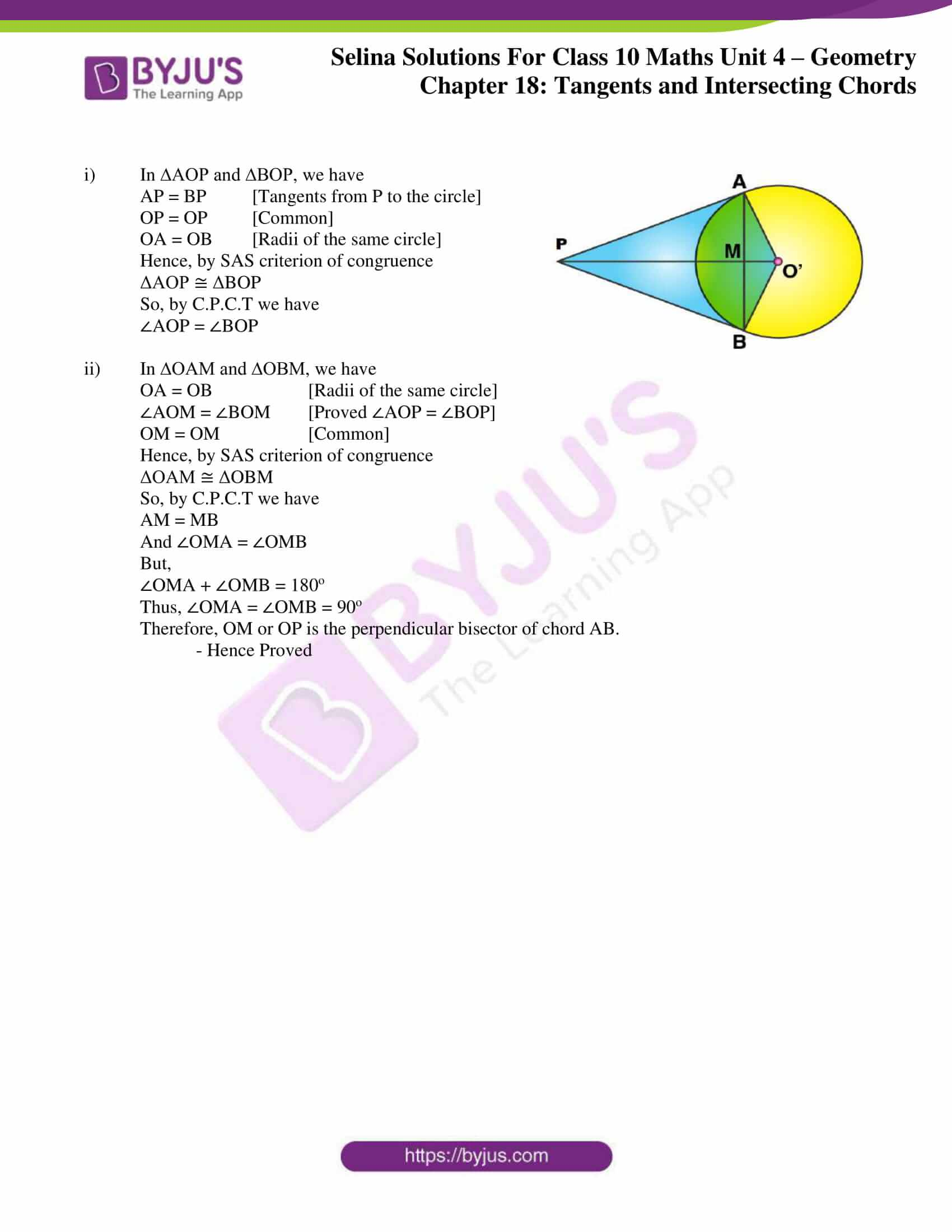### Access Selina Solutions Concise Maths Class 10 Chapter 18 Tangents and Intersecting Chords Exercise 18(A)

1. The radius of a circle is 8cm. Calculate the length of a tangent drawn to this circle from a point at a distance of 10cm from its centre.

Solution: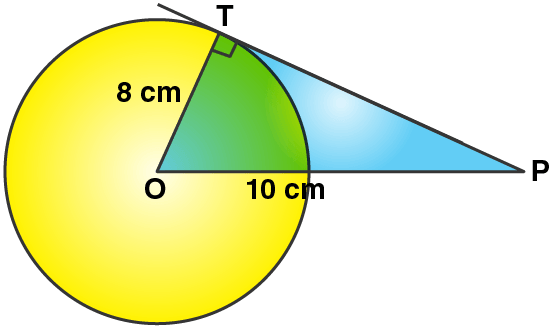Given, a circle with centre O and radius 8 cm.

An external point P from where a tangent is drawn to meet the circle at T.

OP = 10 cm; radius OT = 8 cm

As OT ⊥ PT

In right ∆OTP, we have

OP2 = OT2 + PT2 [By Pythagoras Theorem]

102 = 82 + PT2

PT2 = 100 – 64 = 36

So, PT = 6

Therefore, length of tangent = 6 cm.

2. In the given figure, O is the centre of the circle and AB is a tangent to the circle at B. If AB = 15 cm and AC = 7.5 cm, calculate the radius of the circle.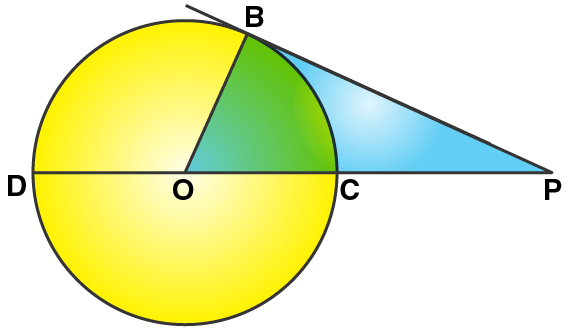Solution:

Given,

AB = 15 cm, AC = 7.5 cm

Let’s assume the radius of the circle to be ‘r’.

So, AO = AC + OC = 7.5 + r

In right ∆AOB, we have

AO2 = AB2 + OB2 [By Pythagoras Theorem]

(7.5 + r)2 = 152 + r2

56.25 + r2 + 15r = 225 + r2

15r = 225 – 56.25

r = 168.75/ 15

Thus,

r = 11.25 cm

3. Two circles touch each other externally at point P. Q is a point on the common tangent through P. Prove that the tangents QA and QB are equal.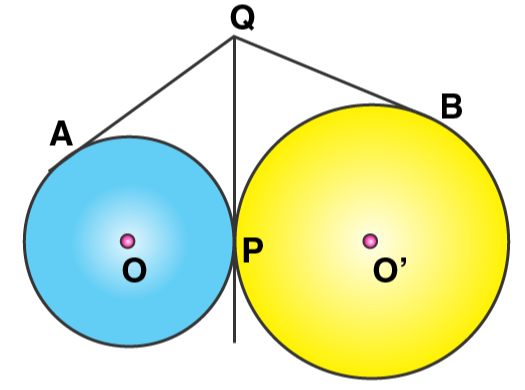Solution:

Let Q be the point from which, QA and QP are two tangents to the circle with centre O

So, QA = QP …..(a)

Similarly, from point Q, QB and QP are two tangents to the circle with centre O’

So, QB = QP ……(b)

From (a) and (b), we have

QA = QB

Therefore, tangents QA and QB are equal.

– Hence Proved

4. Two circles touch each other internally. Show that the tangents drawn to the two circles from any point on the common tangent are equal in length.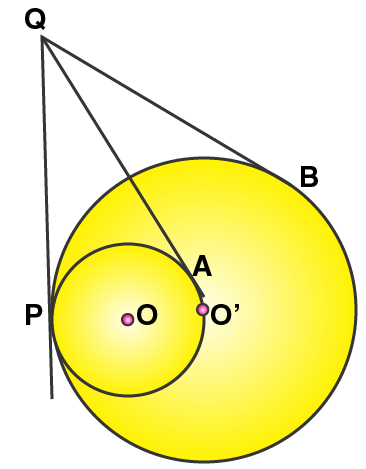Solution:

Let Q be the point on the common tangent from which, two tangents QA and QP are drawn to the circle with centre O.

So, QA = QP ……. (1)

Similarly, from point Q, QB and QP are two tangents to the circle with centre O’

So, QB = QP ……. (2)

From (1) and (2), we have

QA = QB

Therefore, tangents QA and QB are equal.

– Hence Proved

5. Two circles of radii 5 cm and 3 cm are concentric. Calculate the length of a chord of the outer circle which touches the inner.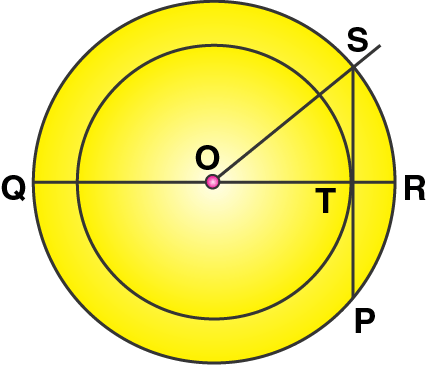Solution:

Given,

OS = 5 cm and OT = 3 cm

In right triangle OST, we have

ST2 = OS2 – OT2

= 25 – 9

= 16

So, ST = 4 cm

As we know, OT is perpendicular to SP and OT bisects chord SP

Hence, SP = 2 x ST = 8 cm

6. Three circles touch each other externally. A triangle is formed when the centers of these circles are joined together. Find the radii of the circles, if the sides of the triangle formed are 6 cm, 8 cm and 9 cm.

Solution:

Let ABC be the triangle formed when centres of 3 circles are joined.

Given,

AB = 6 cm, AC = 8 cm and BC = 9 cm

And let the radii of the circles having centres A, B and C be r1, r2 and r3 respectively.

So, we have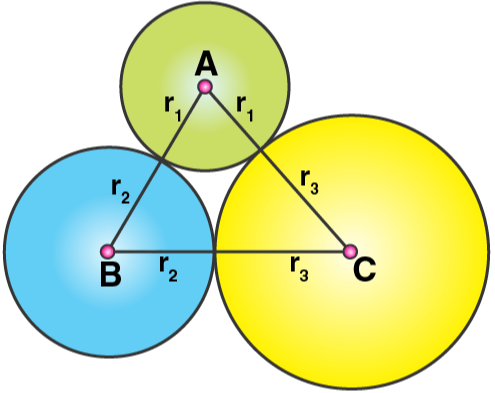r1 + r3 = 8

r3 + r2 = 9

r2 + r1 = 6

Adding all the above equations, we get

r1 + r3 + r3 + r2 + r2 + r1 = 8 + 9 + 6

2(r1 + r2 + r3) = 23

So,

r1 + r2 + r= 11.5 cm

Now,

r1 + 9 = 11.5 (As r2 + r= 9)

r1 = 2.5 cm

And,

r2 + 6 = 11.5 (As r1 + r= 6)

r2 = 5.5 cm

Lastly, r3 + 8 = 11.5 (As r2 + r= 8)

r= 3.5 cm

Therefore, the radii of the circles are r1 = 2.5 cm, r2 = 5.5 cm and r= 3.5 cm.

7. If the sides of a quadrilateral ABCD touch a circle, prove that AB + CD = BC + AD.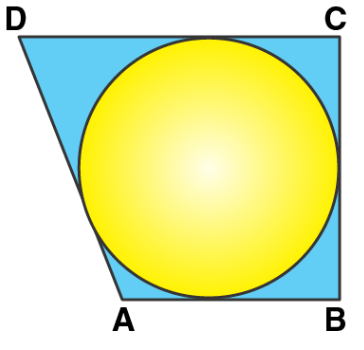Solution: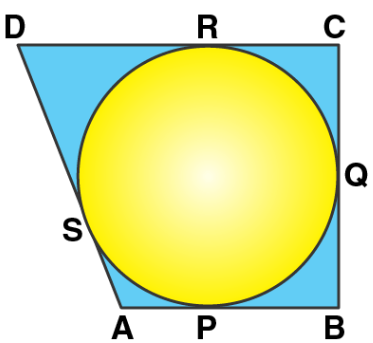Let a circle touch the sides AB, BC, CD and DA of quadrilateral ABCD at P, Q, R and S respectively.

As, AP and AS are tangents to the circle from an external point A, we have

AP = AS ……. (1)

Similarly, we also get

BP = BQ ……. (2)

CR = CQ ……. (3)

DR = DS …….. (4)

Adding (1), (2), (3) and (4), we get

AP + BP + CR + DR = AS + DS + BQ + CQ

AB + CD = AD + BC

Therefore,

AB + CD = AD + BC

– Hence Proved

8. If the sides of a parallelogram touch a circle, prove that the parallelogram is a rhombus.Solution:

Let a circle touch the sides AB, BC, CD and DA of parallelogram ABCD at P, Q, R and S respectively.

Now, from point A, AP and AS are tangents to the circle.

So, AP = AS……. (1)

Similarly, we also have

BP = BQ ……… (2)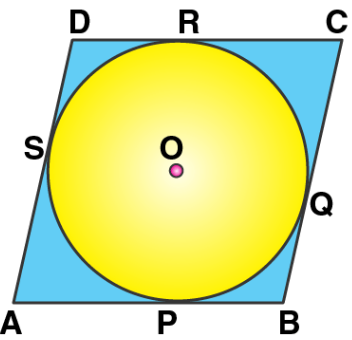CR = CQ ……… (3)

DR = DS ……… (4)

Adding (1), (2), (3) and (4), we get

AP + BP + CR + DR = AS + DS + BQ + CQ

AB + CD = AD + BC

Therefore,

AB + CD = AD + BC

But AB = CD and BC = AD……. (5) [Opposite sides of a parallelogram]

Hence,

AB + AB = BC + BC

2AB = 2 BC

AB = BC …….. (6)

From (5) and (6), we conclude that

AB = BC = CD = DA

Thus, ABCD is a rhombus.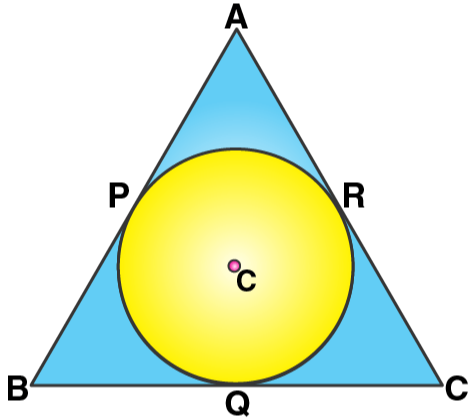9. From the given figure prove that:

AP + BQ + CR = BP + CQ + AR.

Also, show that AP + BQ + CR = ½ x perimeter of triangle ABC.

Solution:

As from point B, BQ and BP are the tangents to the circle

We have, BQ = BP ………..(1)

Similarly, we also get

AP = AR ………….. (2)

And, CR = CQ …… (3)

Adding (1), (2) and (3) we get,

AP + BQ + CR = BP + CQ + AR ……… (4)

Now, adding AP + BQ + CR to both sides in (4), we get

2(AP + BQ + CR) = AP + PQ + CQ + QB + AR + CR

2(AP + BQ + CR) = AB + BC + CA

Therefore, we get

AP + BQ + CR = ½ x (AB + BC + CA)

i.e.

AP + BQ + CR = ½ x perimeter of triangle ABC

10. In the figure, if AB = AC then prove that BQ = CQ.

Solution:

As, from point A

AP and AR are the tangents to the circle

So, we have AP = AR

Similarly, we also have

BP = BQ and CR = CQ [From points B and C]

Now adding the above equations, we get

AP + BP + CQ = AR + BQ + CR

(AP + BP) + CQ = (AR + CR) + BQ

AB + CQ = AC + BQ ….. (i)

But, as AB = AC [Given]

Therefore, from (i)

CQ = BQ or BQ = CQ

11. Radii of two circles are 6.3 cm and 3.6 cm. State the distance between their centers if –

i) they touch each other externally.

ii) they touch each other internally.

Solution:

Given,

Radius of bigger circle = 6.3 cm and of smaller circle = 3.6 cm

i)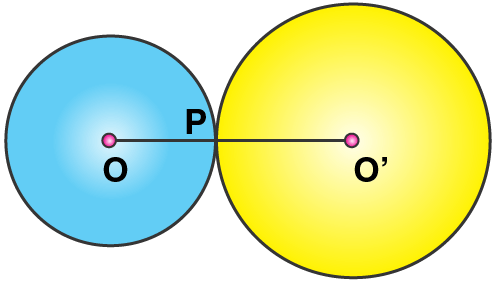When the two circles touch each other at P externally. O and O’ are the centers of the circles. Join OP and O’P.

So, OP = 6.3 cm, O’P = 3.6 cm

Hence, the distance between their centres (OO’) is given by

OO’ = OP + O’P = 6.3 + 3.6 = 9.9 cm

ii)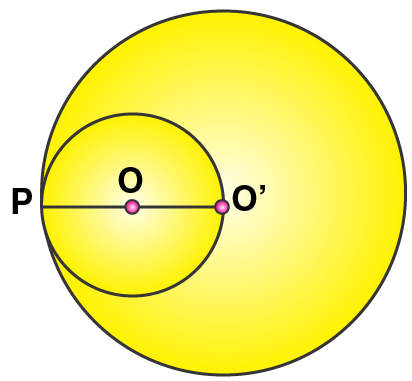When the two circles touch each other at P internally. O and O’ are the centers of the circles. Join OP and O’P

So, OP = 6.3 cm, O’P = 3.6 cm

Hence, the distance between their centres (OO’) is given by

OO’ = OP – O’P = 6.3 – 3.6 = 2.7 cm

12. From a point P outside the circle, with centre O, tangents PA and PB are drawn. Prove that:

i) ∠AOP = ∠BOP

ii) OP is the ⊥ bisector of chord AB.

Solution: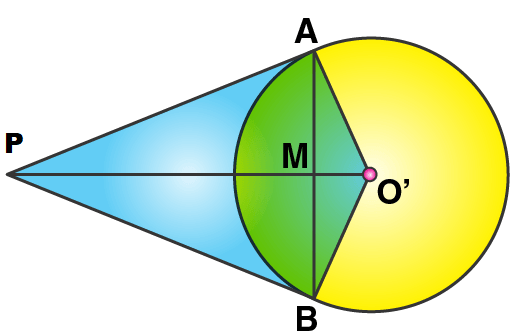i) In ∆AOP and ∆BOP, we have

AP = BP [Tangents from P to the circle]

OP = OP [Common]

OA = OB [Radii of the same circle]

Hence, by SAS criterion of congruence

ΔAOP ≅ ΔBOP

So, by C.P.C.T we have

∠AOP = ∠BOP

ii) In ∆OAM and ∆OBM, we have

OA = OB [Radii of the same circle]

∠AOM = ∠BOM   [Proved ∠AOP = ∠BOP]

OM = OM [Common]

Hence, by SAS criterion of congruence

ΔOAM ≅ ΔOBM

So, by C.P.C.T we have

AM = MB

And ∠OMA = ∠OMB

But,

∠OMA + ∠OMB = 180o

Thus, ∠OMA = ∠OMB = 90o

Therefore, OM or OP is the perpendicular bisector of chord AB.

– Hence Proved